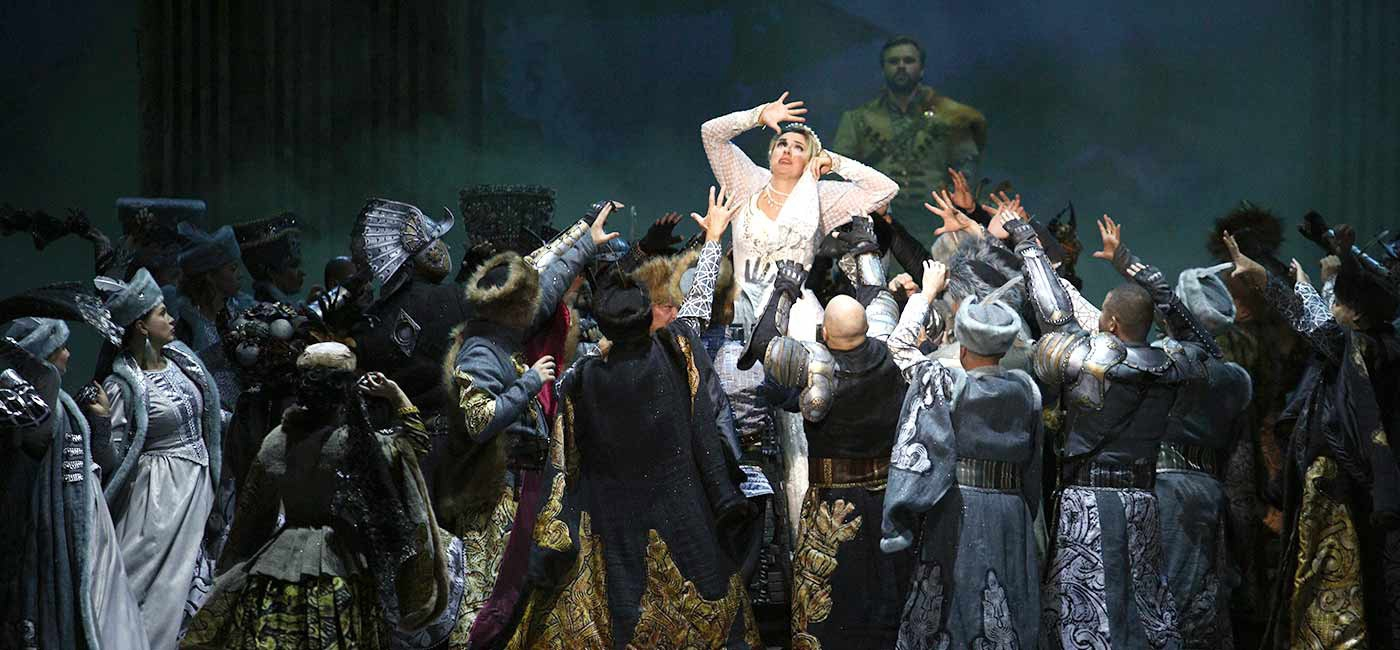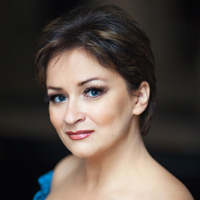# KING STAKHвЂ™S WILD HUNT

opera in two actsGiacomo Puccini

# Tosca

opera in three acts# Elena Tabolich

### ScheduleGrand prize at the International Competition "21st Century Art" (Ukraine, 2009)
Grand prize at the International Competition "21st Century Art" (Italy, 2009)

Elena Tabolich graduated from the Belarusian State Academy of Music in 2003 where she had studied under the tutelage of the Honoured Artist of the Republic of Belarus Lyudmila Kolos.

In 2005 she joined the chorus of the Bolshoi Theatre of Belarus and in 2012 was promoted to soloist.

### REPERTOIRE

Pinocchio,В The FoxВ  В  В  В  В  В  В  В  В  В  В  В  В  В  В  В  В  В  В  В В Pinocchio
Iolanta, BrigittaВ В В В В В В В В В В В В В В В В В В В В В В В В В В В В В В В В В В В В В В В  В  В  Iolanta
AnninaВ В В В В В В В В В В В В В В В В В В В В В В В В В В В В В В В В В В В В В В В В В В В В В В В В В В В В В В В  La traviata
OscarВ В В В В В В В В В В В В В В В В В В В В В В В В В В В В В В В В В В В В В В В В В В В В В В В В В В В В В В В В В  Un ballo in maschera
LuigiaВ В В В В В В В В В В В В В В В В В В В В В В В В В В В В В В В В В В В В В В В В В В В В В В В В В В В В В В В В  Viva la mamma!
Papagena, the First Boy, the First LadyВ В  В  В  В  В  В  Die ZauberflГ¶te
BomВ В В В В В В В В В В В В В В В В В В В В В В В В В В В В В В В В В В В В В В В В В В В В В В В В В В В В В В В В В В  Magic Music
The MouseВ В В В В В В В В В В В В В В В В В В В В В В В В В В В В В В В В В В В В В В В В В В В В  В  В  The Little Tower Chamber
MedeaВ В В В В В В В В В В В В В В В В В В В В В В В В В В В В В В В В В В В В В В В В В В В В В В В В В В В В В В  Metamorphoses ballet
The PigletВ В В В В В В В В В В В В В В В В В В В В В В В В В В В В В В В В В В В В В В В В В В В В В В В В В  Winnie-the-Pooh
Mademoiselle SilberklangВ В В В В В В В В В В В В В В В В В В В В В  В  В  В В  В  Der Schauspieldirektor
ToninaВ В В В В В В В В В В В В В В В В В В В В В В В В В В В В В В В В В В В В В В В В В В В В В В В В В В В В В В  Prima la musica e poi le parole
Prilepa, MashaВ В В В В В В В В В В В В В В В В В В В В В В В В В В В В В В В В В В В В В В В  В  В  The Queen of Spades
The MaidenВ В В В В В В В В В В В В В В В В В В В В В В В В В В В В В В В В В В В В В В В В В В В В В В  The TsarвЂ™s Bride
TanyaВ В В В В В В В В В В В В В В В В В В В В В В В В В В В В В В В В В В В В В В В В В В В В В В В В В В В В В В В В  Doctor Ouchithurts

В

### Partners